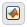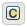## Differences Between MATLAB and C as Action Language Syntax

Stateflow® charts in Simulink® models have an action language property that defines the syntax for state and transition actions. An icon in the lower-left corner of the chart canvas indicates the action language for the chart.

•MATLAB® as the action language.

•C as the action language.

MATLAB is the default action language syntax for new Stateflow charts. To create a chart that uses C as the action language, enter:

`sfnew -c`

### Compare Functionality of Action Languages

This table lists the most significant differences in functionality between the two action languages.

FunctionalityMATLAB as the Action LanguageC as the Action Language

Vector and matrix indexing

One-based indexing delimited by parentheses and commas. For example, `A(4,5)`. See Operations for Vectors and Matrices in Stateflow.

Zero-based indexing delimited by square brackets. For example, `A`. See Operations for Vectors and Matrices in Stateflow.

C constructs:

• Increment and decrement operations `a++` and `a--`

• Assignment operations ```a += b```, `a –= b`, ```a *= b```, and ```a /= b```

• Evaluation operations ```a != b``` and `!a`

• Binary operations `a %% b`, `a >> b`, ```a << b```, `a & b`, and `a | b`

• Comment markers `//` and `/* */`

Auto-correction to MATLAB syntax. For example, `a++` is corrected to `a = a+1`. See Auto Correction When Using MATLAB as the Action Language.

Supported. See Operations for Stateflow Data.

Conditional and loop control statements in state actions

Supported. For example, you can use `if`, `for`, and `while` statements in state actions. See Loops and Conditional Statements.

Not supported. For conditional and loop patterns, use graphical functions instead. See Reuse Logic Patterns by Defining Graphical Functions.

Format of transition actions

Auto-correction encloses transition actions with braces `{}`. See Transition Actions.

Not required to enclose transition actions with braces `{}`. See Transition Actions.

Ordering of parallel states

Explicit ordering only. See Execution Order for Parallel States.

Explicit or implicit ordering. See Execution Order for Parallel States.

Variable-size data

Modify variable-size chart data in state and transition actions. For more information, see Variable-Size Data in Charts That Use MATLAB as the Action Language.

Modify variable-size chart data by using:

• MATLAB functions

• Truth tables that use MATLAB as the action language

All computations with variable-size data must occur inside these functions, and not directly in states or transitions. For more information, see Variable-Size Data in Charts That Use C as the Action Language.

Fixed-point constructs:

• Special assignment operator `:=`

• Context-sensitive constants such as `4.3C`

Not supported.

Complex data

Use complex number notation `a + bi` or the `complex` operator. See Operations for Complex Data in Stateflow.

Use the `complex` operator. Complex number notation is not supported. See Operations for Complex Data in Stateflow.

Data type propagation

Follows MATLAB typing rules. For example, adding data of type `double` to data of type `int32` results in data of type `int32`.

Follows C typing rules. For example, adding data of type `double` to data of type `int32` results in data of type `double`.

Explicit type cast operations

Use one of these casting forms:

• MATLAB type conversion function. For example, `single(x)`.

• `cast` function with a type keyword. For example, `cast(x,"int8")`.

• `cast` function with the `"like"` keyword. For example, `cast(x,"like",z)`.

The `type` operator is not supported. See Type Cast Operations.

Use one of these casting forms:

• MATLAB type conversion function. For example, `uint16(x)`.

• `cast` function with the `type` operator. For example, `cast(x,type(z))`.

Type keywords for the `cast` function are not supported. See Type Cast Operations.

Scalar expansion

Not supported.

Supported. See Assign Values to All Elements of a Matrix.
String data

Use double quotes (`"`...`"`) as delimiters. See Manage Textual Information by Using Strings.

Use double (`"`...`"`) or single quotes (`'`...`'`) as delimiters. See Manage Textual Information by Using Strings.

Specification of data properties:

• First index

• Save final value to base workspace

• Units

Not supported.

Scope of data in graphical, truth table, and MATLAB functions

`Constant`, `Parameter`, `Input`, `Output``Local`, `Constant`, `Parameter`, `Input`, `Output`, `Temporary`

Dot notation for specifying states, local data, message, and local events inside MATLAB functions

Supported. See Identify Data by Using Dot Notation.

Not supported.

Custom code functions and variables

Behavior depends on the Import Custom Code configuration parameter.

• When you enable Import Custom Code, both custom code functions and variables are supported in states and transitions (default).

• When you disable Import Custom Code, only custom code functions are supported. Use the `coder.ceval` (MATLAB Coder) function.

See Reuse Custom Code in Stateflow Charts and Import custom code (Simulink).

Custom code functions and variables are supported in states and transitions.

Structure parameters

Tunable and nontunable parameters are supported.

Only tunable parameters are supported.

Use of global `fimath` object

Supported.

Not supported.

### Guidelines for Using MATLAB as the Action Language

#### Use one-based indexing for vectors and matrices

One-based indexing is consistent with MATLAB syntax. For more information, see Indexing Notation.

#### Use parentheses instead of brackets to index into vectors and matrices

This statement is valid:

`a(2,5) = 0;`

This statement is not valid:

`a = 0;`

#### Use the MATLAB format for comments

Use `%` to specify comments in states and transitions for consistency with MATLAB. For example, the following comment is valid:

`% This is a valid comment in the style of MATLAB`

C style comments, such as `//` and `/* */`, are auto-corrected to use %.

#### Enclose transition actions with braces

This transition label contains a valid transition action:

``E [x > 0] / {x = x+1;}``

This transition label is incorrect, but is auto-corrected to the valid syntax.

``E [x > 0] / x = x+1;``

#### Do not use control flow logic in condition actions and transition actions

Control flow logic (such as `if`, `switch`, `for`, and `while` statements) is supported only in state actions. Use of control flow logic in condition actions or transition actions, result in a syntax error.

#### Do not declare global or persistent variables in state actions

The keywords `global` and `persistent` are not supported in state actions.

#### Assign an initial value to local and output data

When using MATLAB as the action language, data read without an initial value causes an error.

#### Include a type prefix for identifiers of enumerated values

The identifier `TrafficColors.Red` is valid, but `Red` is not.

#### To generate code from your model, use MATLAB language features supported for code generation

Otherwise, use `coder.extrinsic` to call unsupported functions, which gives the functionality that you want for simulation, but not in the generated code. For a list of supported features and functions, see Language, Function, and Object Support (Simulink).